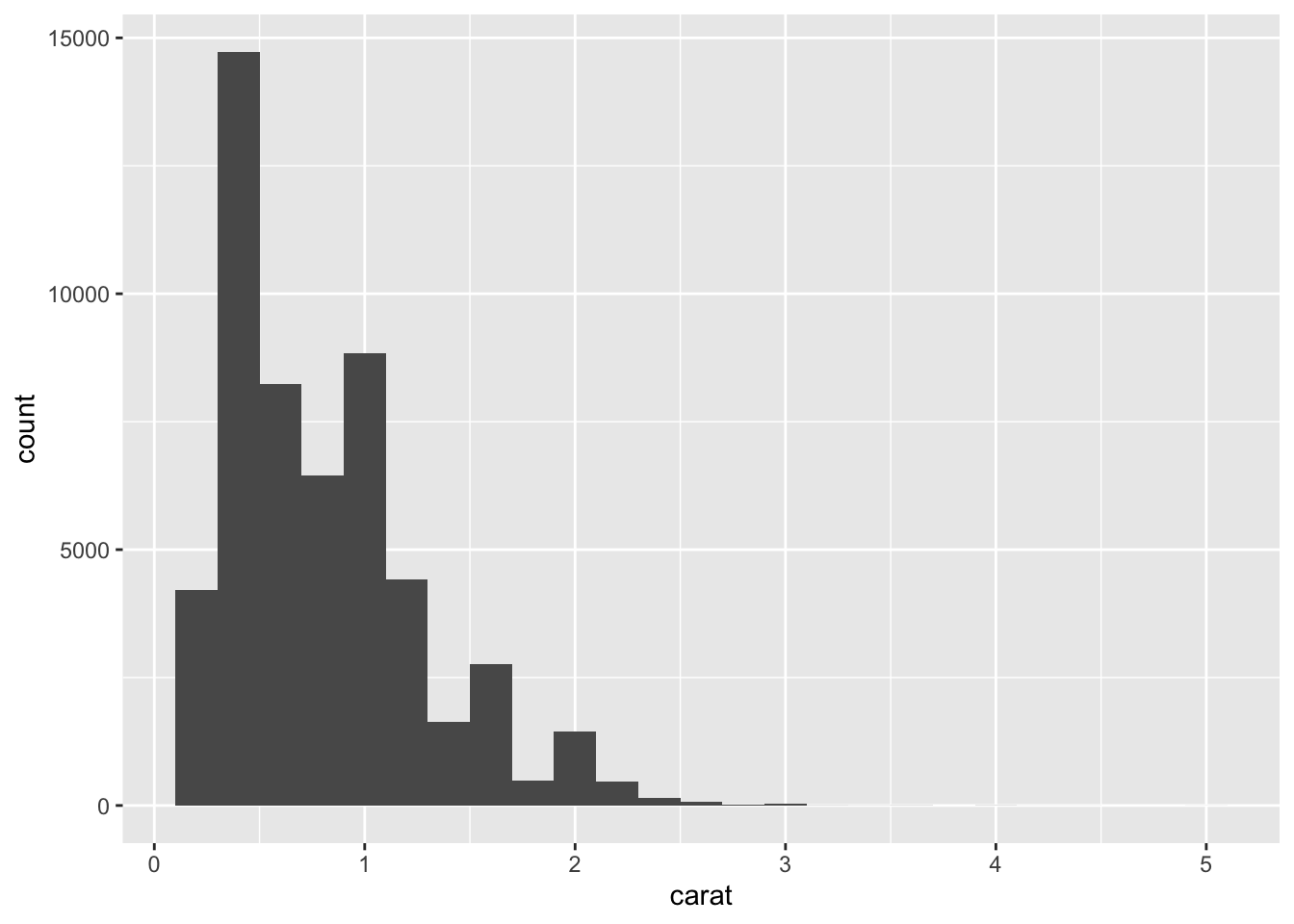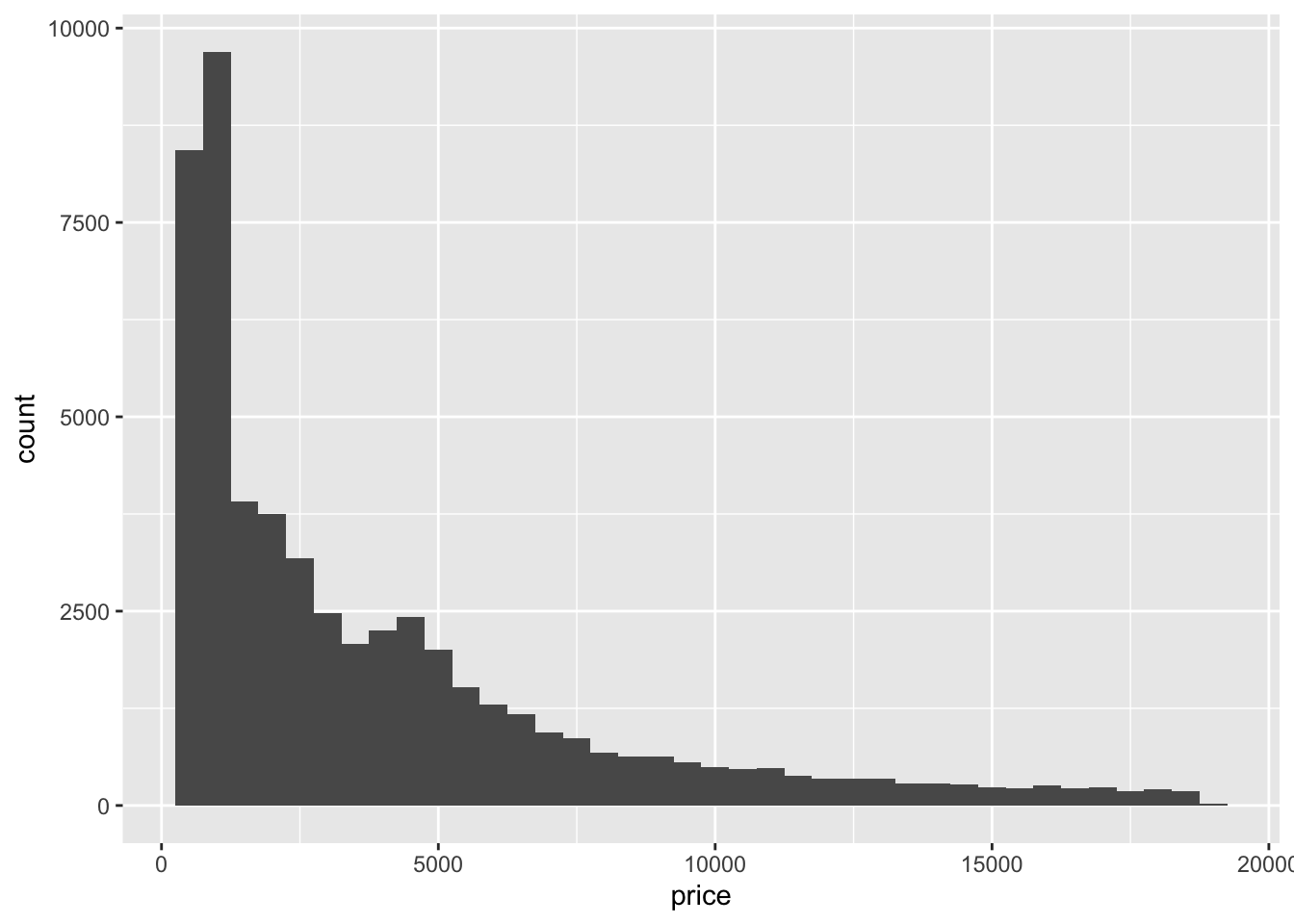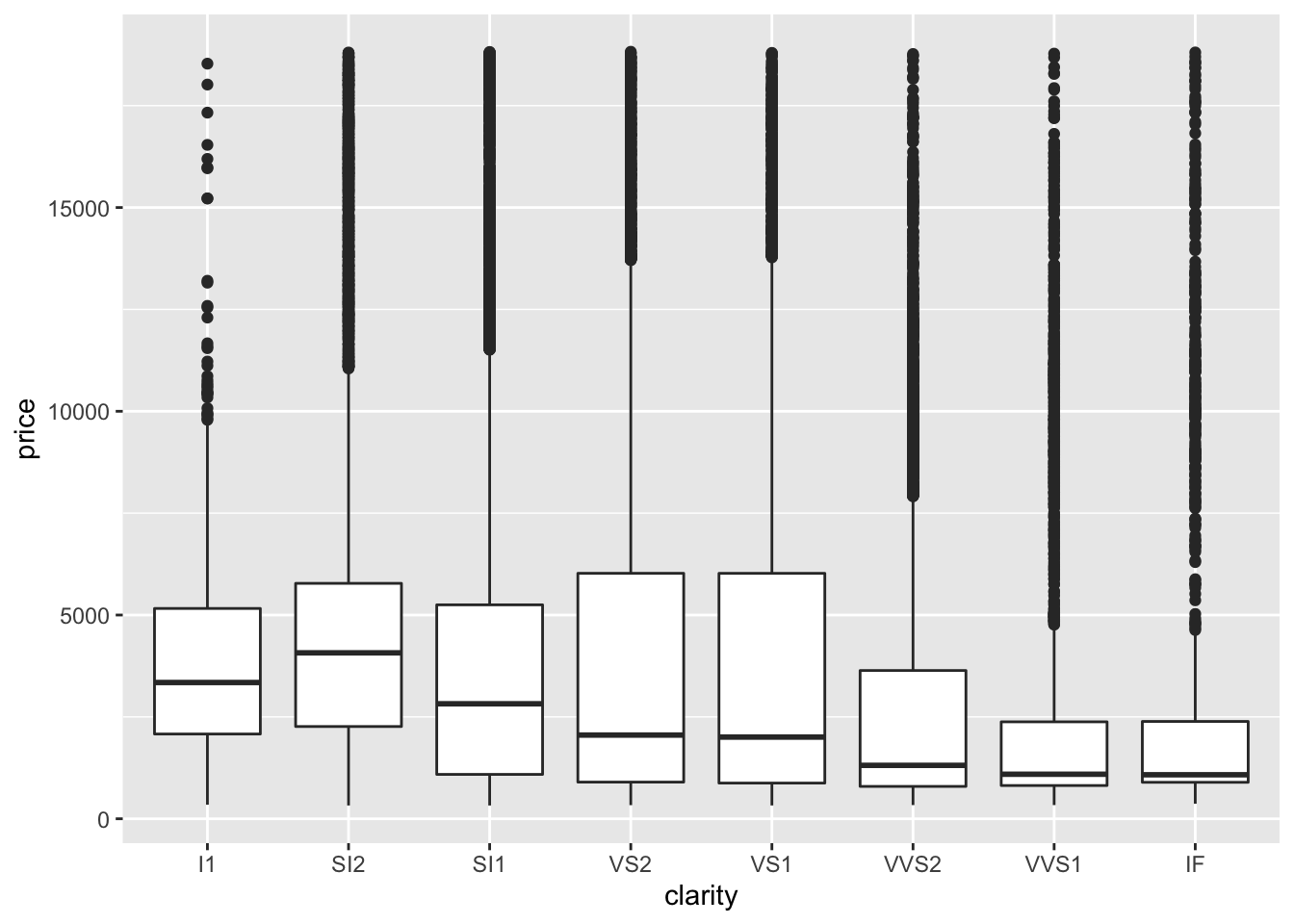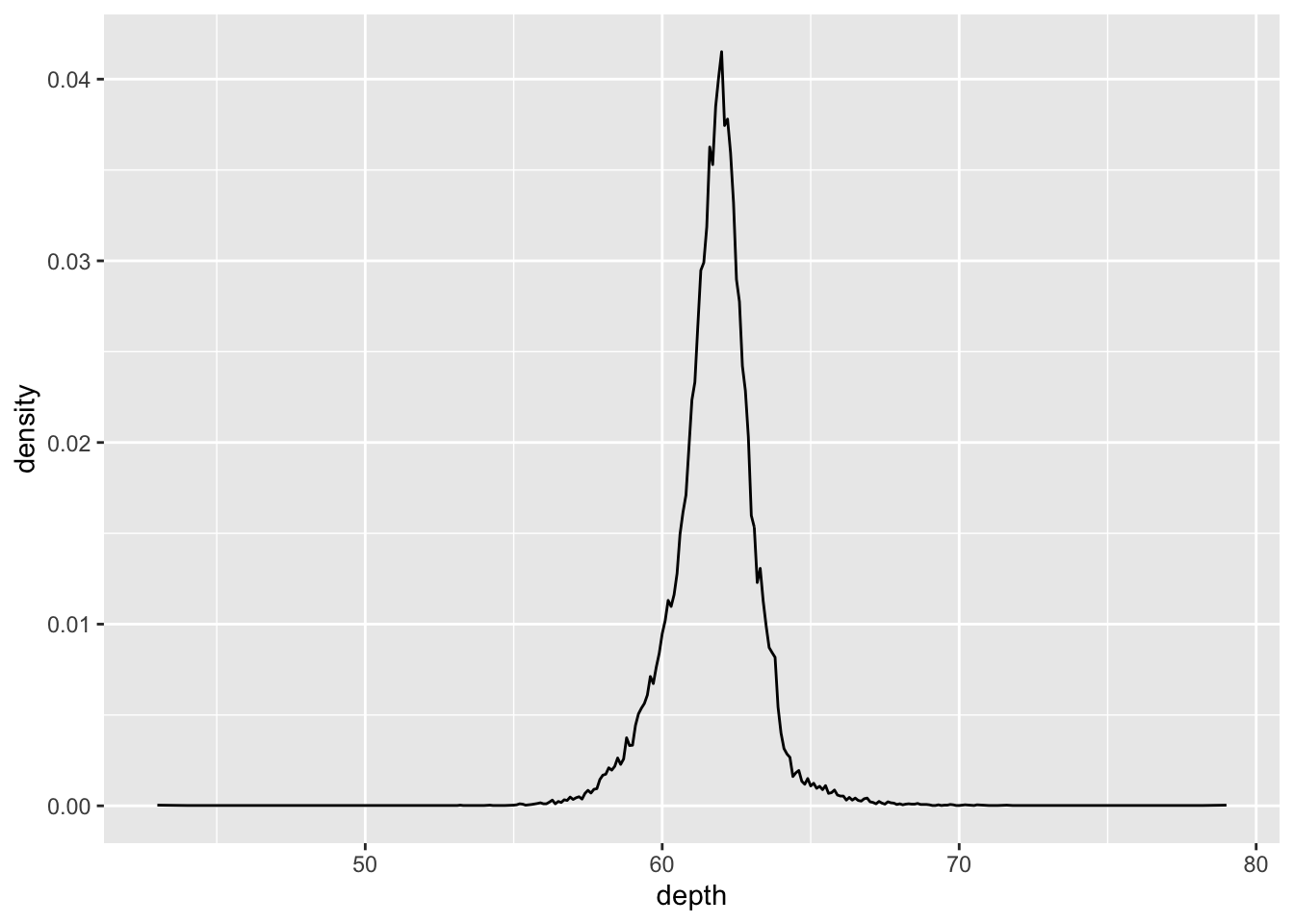# 5 Statistical Summaries

## 5.1 Exercises

1. What binwidth tells you the most interesting story about the distribution of `carat`?

``````diamonds %>%
ggplot(aes(carat)) +
geom_histogram(binwidth = 0.2)``````• Highly subjective answer, but I would go with 0.2 since it gives you the right amount of information about the distribution of `carat`: right-skewed.

2. Draw a histogram of `price`. What interesting patterns do you see?

``````diamonds %>%
ggplot(aes(price)) +
geom_histogram(binwidth = 500)``````• It’s skewed to the right and has a long tail. Also, there is a small peak around 5000 and a huge peak around 0.

3. How does the distribution of `price` vary with `clarity`?

``````diamonds %>%
ggplot(aes(clarity, price)) +
geom_boxplot()``````• The range of prices is similar across clarity and the median and IQR vary greatly with clarity.

4. Overlay a frequency polygon and density plot of `depth`. What computed variable do you need to map to `y` to make the two plots comparable? (You can either modify `geom_freqpoly()` or `geom_density()`.)

``````diamonds %>%
count(depth) %>%
mutate(sum = sum(n),
density = n / sum) %>%
ggplot(aes(depth, density)) +
geom_line()``````• Say you start off with the count of values in `depth` and you plot `geom_freqpoly()`. Then, you would want to divide each count by the total number of points to get density. This would get you the y variable needed for `geom_density()`